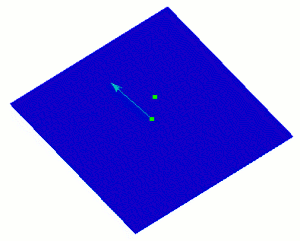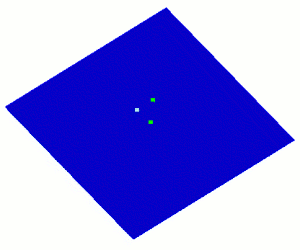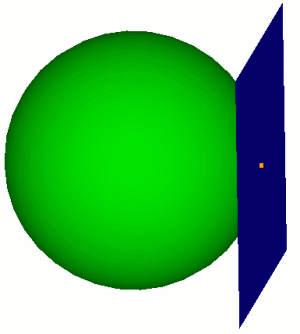# Specifying a Plane

Some commands require a specified plane (such as sweep curve target) for the command. The following options determine a plane specification:

The following options apply to all of the plane specifications listed above:

## Location and Normal Vector

{Location|Vertex|Node} <origin> Direction <normal>

The first way to specify a plane is to specify a starting point and a direction vector:

draw plane location 1 2 3 direction 0 1 1
draw plane vertex 1 direction
tangent at curve 1Figure 1. Specifying a plane with a location and surface normal

To see the options for location specification, see Specifying a Location. Direction options can be found at Specifying a Direction.

## Location and Two Vectors on the Plane

{Location|Vertex|Node} <origin> Direction <vec on plane> Direction <vec on plane>

It is also possible to select an origin point and 2 direction vectors on the plane..

Figure 2. Specifying a plane with a point and 2 in-plane vectors

## Two Locations and Vector on the Plane

{Location|Vertex|Node} <2 locations> Direction <vector on the plane>

You can also specify 2 locations and 1 direction on the plane to define the plane.

draw plane vertex 1 2 direction 0 1 1Figure 3. Specifying 2 locations and 1 direction on the plane

## Three Points on the Plane

{Location|Vertex|Node} <3 locations>

A plane can be defined by three locations, vertices, or nodes. The locations are specified using Location Specification.

draw plane vertex 1 2 3
draw plane vertex 1 2 location 3 4 5Figure 4. A plane specified by three points

## Plane defined by a Surface

Surface <id> [At Location <loc>]

The surface option uses and existing surface to define the plane. If it is not a planar surface, the optional location specifier can be used to find the tangent plane of a specific point on the surface.

draw plane surface 1 at location 4 0 0Figure 5. Specifying a Tangent plane to a Surface

## Plane Normal to a Curve

[Normal To] Curve <id> [loc on curve options]

The Normal to Curve option allows you to define a plane by using an existing curve. The direction of the curve will define the surface normal of the new plane. The optional location argument specifies which point to use on the curve if it is not a straight curve. If no location is specified the plane will originate at the midpoint of the curve. See Specifying a Location on a Curve for more information on location options.

brick x 10
subtract body 2 from 1
webcut body 1 xplane
draw plane normal to curve 30Figure 6. Draw Plane Normal to Curve

## Plane Defined by a Non-linear curve

Arc Curve <id>

A plane can be defined by a single curve, provided that curve is not linear.

draw plane arc curve 2

## Plane Defined by a two linear curves

Linear Curve <id> <id>

A plane can be defined by a two linear curves, provided that the curves are not co-linear.

brick x 10
draw plane linear curve 2 3

## Normal Vector and Coefficient

Direction <Normal> Coefficient <val>

The direction and coefficient option allows you to specify a plane based on a vector and an offset from the origin. The Coefficient argument specifies how far to offset the plane from the origin

draw plane direction 1 2 3 coefficient 3

## Coordinate Plane

X|Xplane|Yz|Zy|Y|Yplane|Zx|Xz|Z|Zplane|Xy|Yx

A plane can be defined from any coordinate plane or combination thereof. The coordinate planes will pass through the origin unless optional specifiers are included.

draw plane xplane
webcut volume 1 plane xz

## Last Location Used

Last

The last option will return the plane most recently used in a command. Last locations do not carry over from CUBIT session to CUBIT session. The last location defaults to (0, 0, 0) if no location has been used during the session.

The following options apply to all of the plane specification methods described above.

• [Offset <val>]
• [Move {<xval yval zval>| {Dx|X|Dy|Y|Dz|Z} <val> | Direction {options} [Distance <val>]]
• [[To] Location {options}]
• [Spin [About] Axis {options} Angle <ang>]]

A offset value will offset the plane in the direction of the surface normal.

The move option will displace the plane in the specified directions by the specified distance. The direction options are outlined on Specifying a Direction.

The location option will move the plane to a specified location without rotating it. See Specifying a Location for location options.

The spin option will rotate the plane around an axis. See Specifying an Axis for axis options.

## Previewing a Plane

The ability to preview a plane prior to creating the plane or using it in a command is possible with the following commands:

Draw Plane (options) [Graphics | {[Intersecting] {Body|Volume} <id_range>] [ [Extended] {Percentage|Absolute} <val>]}] [Color 'color_name']

The options for specifying a plane are described above in the section on Plane Specification. By default, the commands draw the plane just large enough to intersect the bounding box of the entire model with minimum surface area. Optionally, you can give a list of bodies to intersect for this calculation. You can also extend the size of the surface by either a percentage distance or an absolute distance of the minimum area size. The default color is blue, but you can specify a different one. See the Appendix of the CUBIT Users Guide for available colors in CUBIT.

## Preview a Cylindrical Plane

The ability to preview a cylindrical plane is possible with the following command:

Draw Cylinder Radius <val> Axis {x|y|z|Vertex <id_1> Vertex <id_2> | <xyz values>} [Center <x_val> <y_val> <z_val>] [[Intersecting] Body <id_range>] [Extended Percentage|Absolute <val>] [Color 'color_name']

The cylinder is defined by a radius and the cylinder axis. The axis is specified as a line corresponding to a coordinate axis, the normal to a specified surface, two arbitrary points, or an arbitrary point and the origin. The center point through which the cylinder axis passes can also be specified.

By default, the commands draw the cylinder just large enough to just intersect the bounding box of the entire model. Optionally, you can give a list of bodies to intersect for this calculation. You can also extend the length of the cylinder by either a percentage distance or an absolute distance of the cylinder length. The default color is blue, but you can specify a different one. See the Appendix of the CUBIT Users Guide for available colors in CUBIT.